# MultiscaleDTM

Ilich, A. R., Misiuk, B., Lecours, V., & Murawski, S. A. (2023). MultiscaleDTM: An open-source R package for multiscale geomorphometric analysis. Transactions in GIS, 27(4). https://doi.org/10.1111/tgis.13067

## Purpose

This R package calculates multi-scale geomorphometric terrain attributes from regularly gridded digital terrain models (DTM; i.e. elevation or bathymetry rasters) via a specified window as described in Ilich et al. (2023).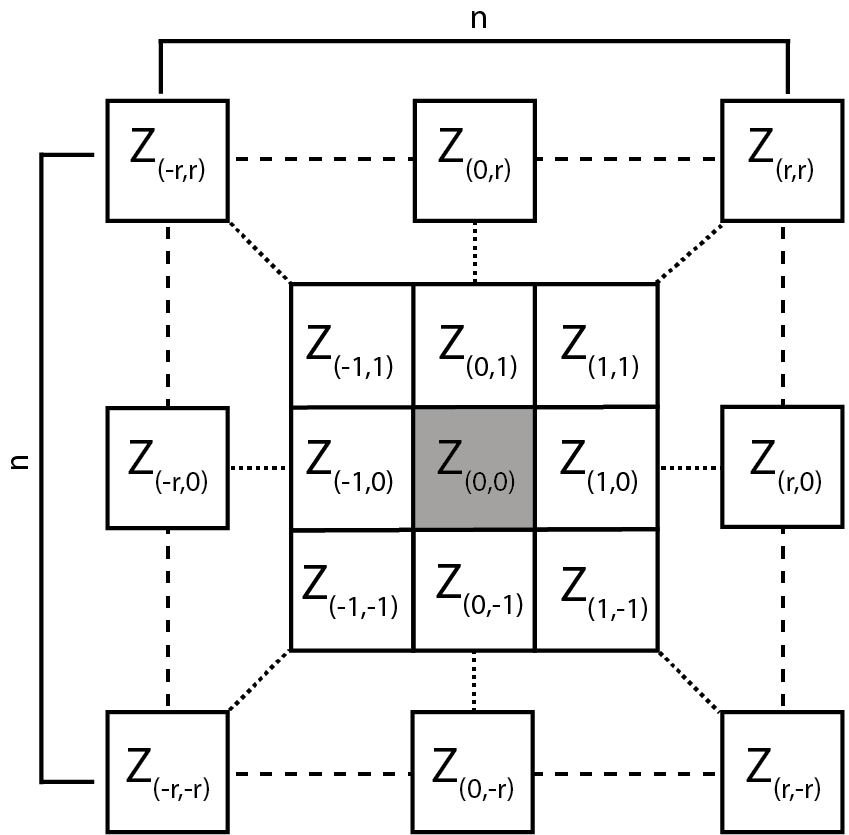Figure adapted from Wilson et al. (2007)

The package can be installed from CRAN using install.packages("MultiscaleDTM") or the development version can be installed from github using the code remotes::install_github("ailich/MultiscaleDTM"). If you are using Windows, you may need to install Rtools using the instructions found here). To install from github you must already have the remotes package installed, which can be installed using install.packages("remotes")

This package relies on the terra package for handling of spatial raster data.

## Main Functions

### Slope, Aspect and Curvature

• SlpAsp calculates multi-scale slope and aspect according to Misiuk et al (2021) which is a modification of the traditional 3 x 3 slope and aspect algorithms (Fleming and Hoffer, 1979; Horn et al., 1981; Ritter, 1987). This algorithm only considers a subset of cells within the focal window, specifically the four cells on the edge of the focal window directly up, down, left, and right of the focal cell for the “rook” case and an additional four corner cells for the “queen” case.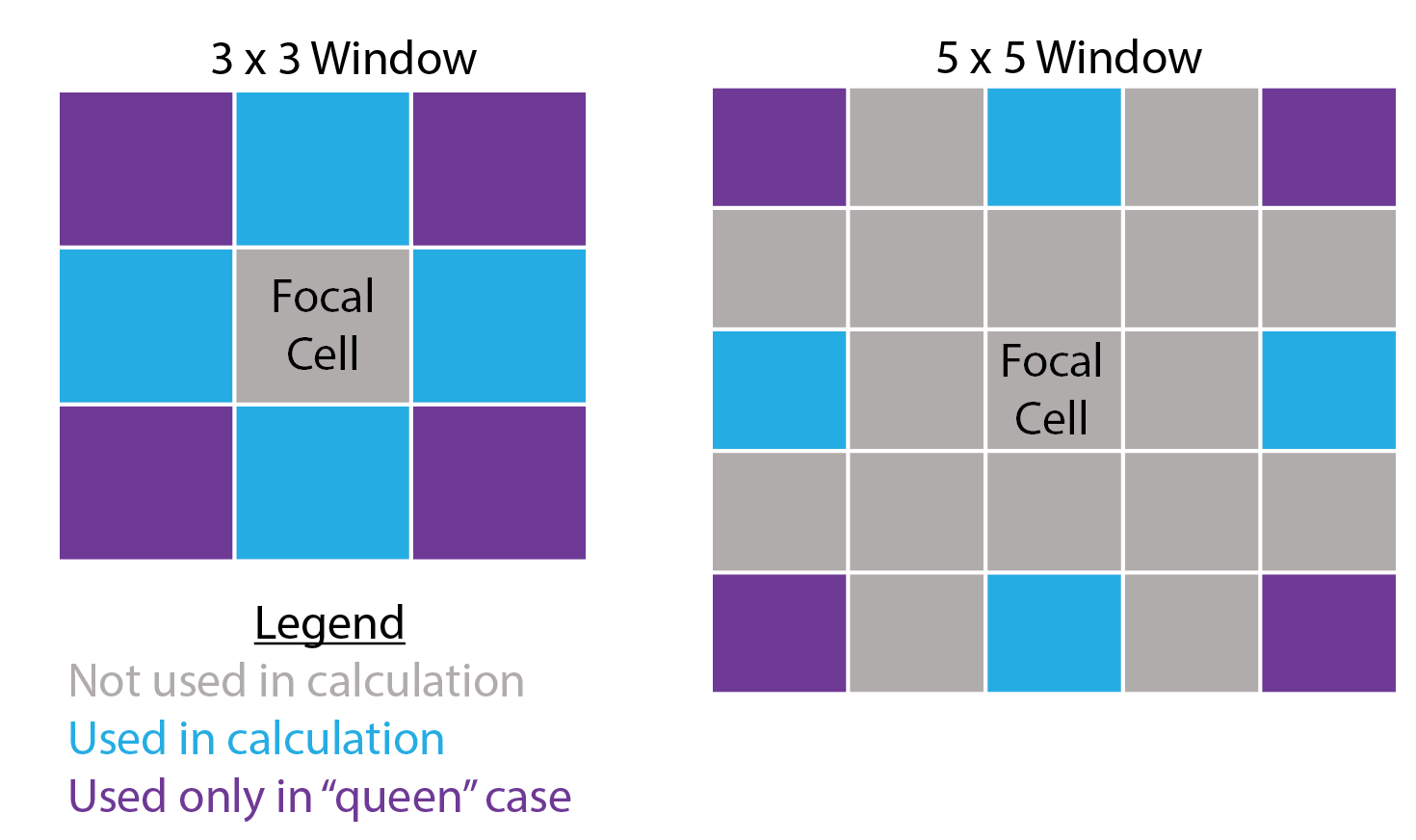• Qfit calculates slope, aspect, curvature, and morphometric features by fitting a quadratic surface to the focal window using ordinary least squares using the equation shown below where a-f are regression parameters, Z is the elevation/depth, X is the east/west coordinates in the focal window relative to the focal cell, and Y is the north/south coordinates in the focal window relative to the focal cell (Evans, 1980; Wilson et al., 2007; Wood, 1996). The morphometric features algorithm has been modified to use more robust measures of curvature based on the suggestions of Minár et al. (2020).

$Z = aX^2 + bY^2 +cXY+ dX +eY +f$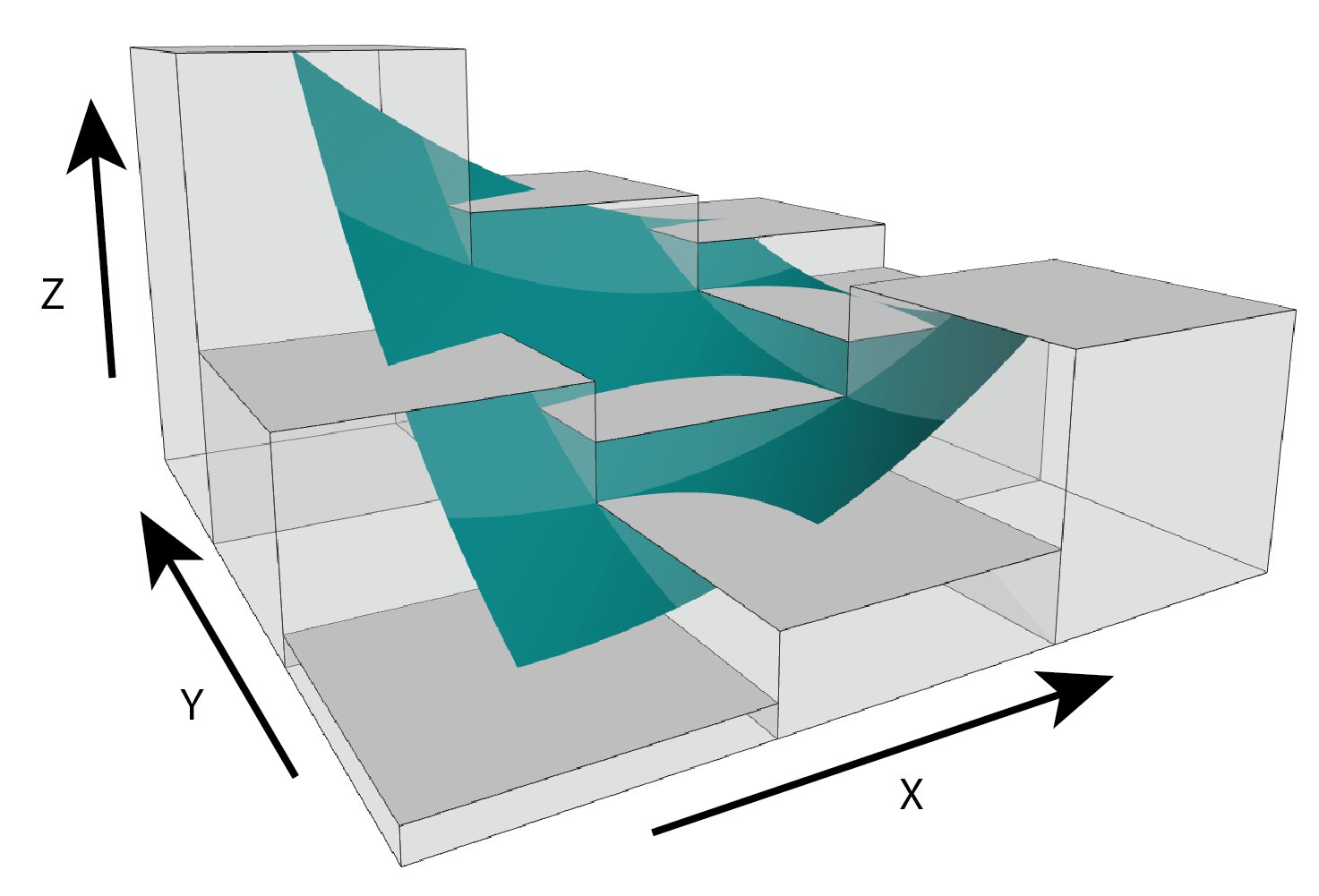Figure adapted from Walbridge et al., (2018)

### Roughness

• VRM - Vector ruggedness measure (Sappington et al. 2007) quantifies roughness by measuring the dispersion of vectors normal to the terrain surface. This is accomplished by calculating the local (3 x 3 cell) slope and aspect, and constructing unit vectors normal to each cell in the DTM. These unit vectors are then decomposed into their corresponding x, y, and z components (i.e. the x, y, and z coordinates of the head of the vector relative to its origin) and used in the following equation (note: N is the number of cells in the window). VRM ranges from zero to one, representing completely smooth to rough surfaces, respectively. .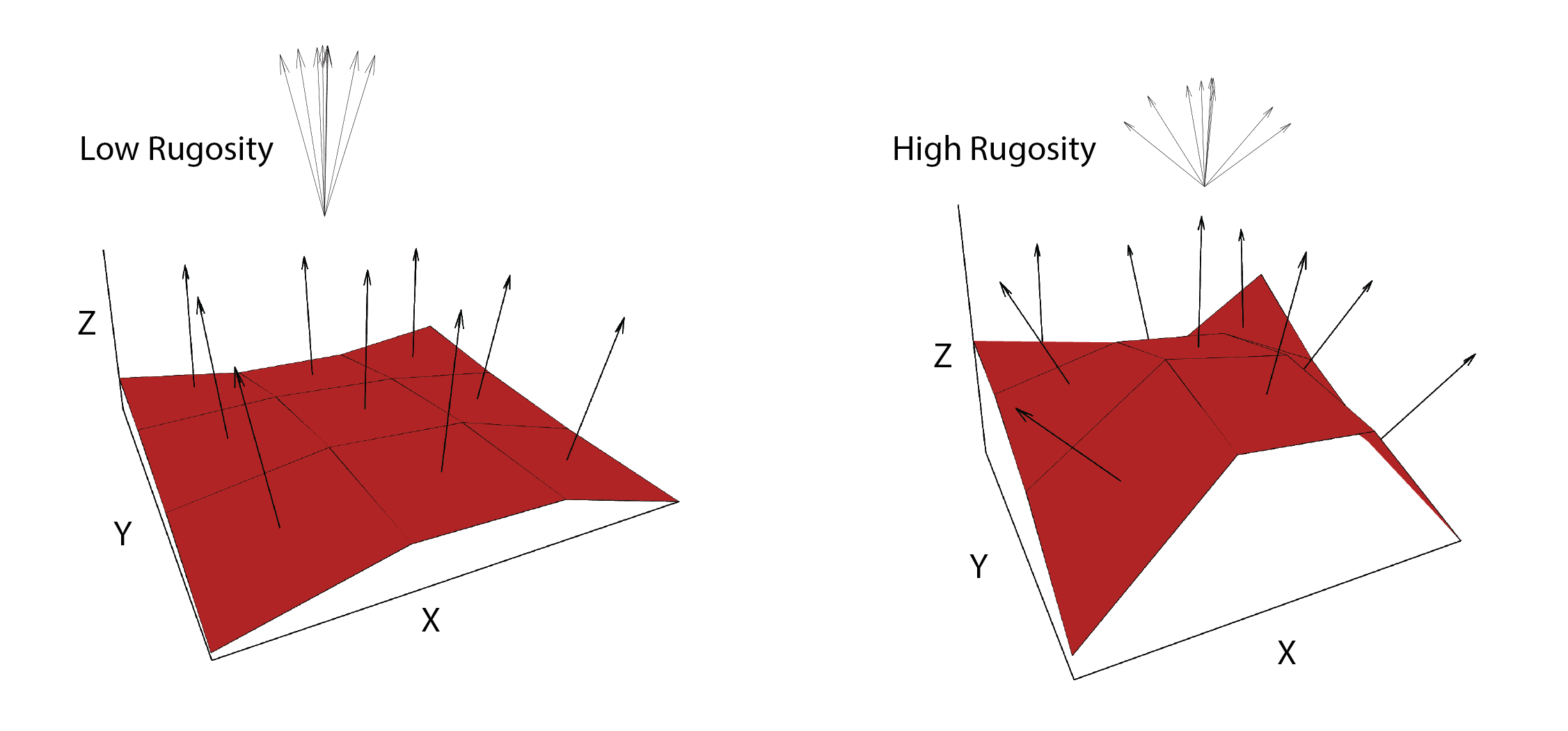Figure adapted from Sappington et al. (2007)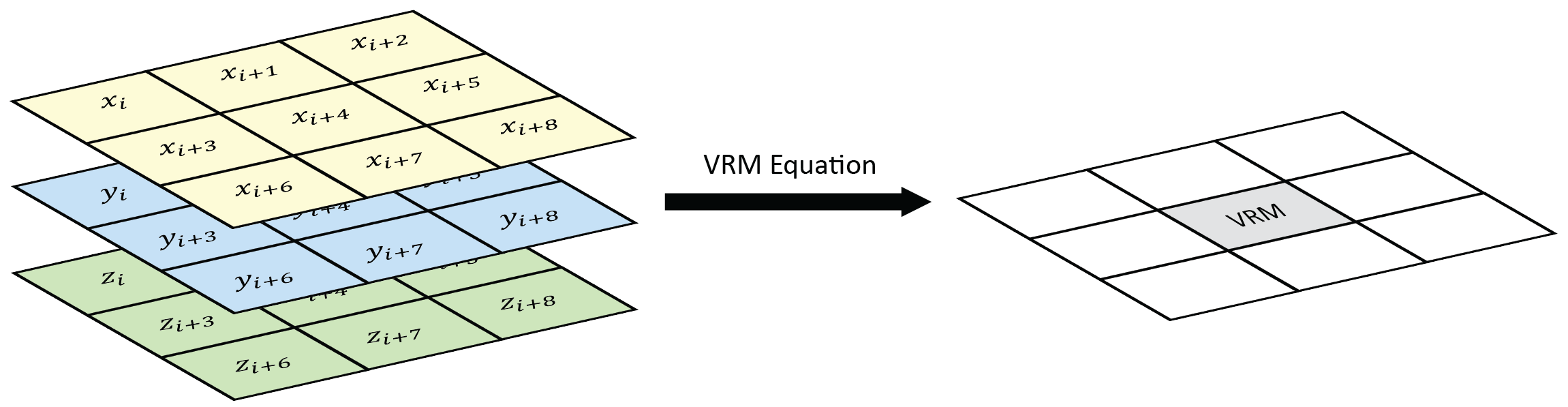$\text{VRM} = 1- \frac{\sqrt{\bigg(\sum x\bigg)^2+\bigg(\sum y\bigg)^2+\bigg(\sum z\bigg)^2}}{N}$
$x = sin(\text{slope})*sin(\text{aspect})$
$y=sin(\text{slope})*cos(\text{aspect})$
$z=cos(\text{slope})$
• SAPA - Calculates the Surface Area to Planar Area (Jenness, 2004). Rougher surfaces will have a greater surface area to planar area ratio, and perfectly smooth surfaces will have a value of 1. This is a 3D analog to the classical “chain-and-tape” method, which calculates roughness as the ratio of the contoured distance (chain length) and linear distance (tape measure distance; Risk, 1972). Additionally, planar area can be corrected for slope by dividing the product of the x and y resolution by the cosine of slope (Du Preez 2015). The metric by Jenness (2004) and De Preez (2015) works at the per cell level (1x1 cell). This function generalizes this method to multiple scales by summing the surface areas within the focal window and adjusting the planar area of the focal window using multi-scale slope (Ilich et al., 2023).
• SurfaceArea - Calculate the surface area of each grid cell (Jenness, 2004). This is accomplished by connecting a focal cell to its immediate neighbors to create 8 large triangles. These large triangles are then trimmed back to the extent of the focal cell using the principle of similar triangles, and then the area of those 8 smaller triangles are calculated and summed to estimate the surface area of the focal pixel. This is used within SAPA.